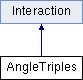ParM  parm A molecular dynamics library
AngleTriples Class Reference

#include <interaction.hpp>

Inheritance diagram for AngleTriples:## Public Member Functions

AngleTriples (vector< AngleGrouping > triples=vector< AngleGrouping >())

bool add (AngleGrouping b, bool replace=true)
Add a triple of atoms. More...

bool add (flt k, flt x0, AtomID a1, AtomID a2, AtomID a3, bool replace=true)

bool add (flt k, AtomID a1, AtomID a2, AtomID a3, bool replace=true)
Add a triple of atoms with the current angle. More...

void add_forced (AngleGrouping b)

flt energy (Box &box)
Potential energy due to this Interaction. More...

flt pressure (Box &box)
Partial pressure due to this Interaction. More...

void set_forces (Box &box)

flt set_forces_get_pressure (Box &box)
Set forces (Atom.f) and return $$P = \sum_{\left<i,j \right>} \vec r_{ij} \cdot \vec F_{ij}$$ at the same time (see pressure()). More...

uint size () const

flt mean_dists () const

flt std_dists () constPublic Member Functions inherited from Interaction
virtual Matrix stress (Box &box)
The force-moment tensor for the current simulation: More...

virtual ~Interaction ()

## Static Protected Member Functions

static Vec diff (Vec r1, Vec r2)

## Protected Attributes

vector< AngleGroupingtriples

## Constructor & Destructor Documentation

 AngleTriples::AngleTriples ( vector< AngleGrouping > triples = vector() )

## Member Function Documentation

 bool AngleTriples::add ( AngleGrouping b, bool replace = true )

Add a triple of atoms.

If "replace", a previous triple found will be replaced by the new triple. If not "replace" and that triple of atoms is already inserted, an error will be thrown.

 bool AngleTriples::add ( flt k, flt x0, AtomID a1, AtomID a2, AtomID a3, bool replace = true )
inline
 bool AngleTriples::add ( flt k, AtomID a1, AtomID a2, AtomID a3, bool replace = true )

Add a triple of atoms with the current angle.

 void AngleTriples::add_forced ( AngleGrouping b )
inline
 static Vec AngleTriples::diff ( Vec r1, Vec r2 )
inlinestaticprotected
 flt AngleTriples::energy ( Box & box )
virtual

Potential energy due to this Interaction.

Implements Interaction.

 flt AngleTriples::mean_dists ( ) const
 flt AngleTriples::pressure ( Box & box )
inlinevirtual

Partial pressure due to this Interaction.

$$P = \sum_{\left<i,j \right>} \vec r_{ij} \cdot \vec F_{ij}$$, or equivalently $$P = \sum_i \vec r_i \cdot \vec F_i$$

Note that the full pressure involves all interactions and temperature, and needs to be normalized by $$\frac{1}{dV}$$ where $$d$$ is the number of dimensions and $$V$$ is the volume.

Implements Interaction.

 void AngleTriples::set_forces ( Box & box )
virtual

Implements Interaction.

 flt AngleTriples::set_forces_get_pressure ( Box & box )
inlinevirtual

Set forces (Atom.f) and return $$P = \sum_{\left<i,j \right>} \vec r_{ij} \cdot \vec F_{ij}$$ at the same time (see pressure()).

Reimplemented from Interaction.

 uint AngleTriples::size ( ) const
inline
 flt AngleTriples::std_dists ( ) const

## Member Data Documentation

 vector AngleTriples::triples
protected

The documentation for this class was generated from the following files: